# College Chemistry : Buffers

## Example Questions

### Example Question #1 : Buffers

Which of the following acid and base pairs are capable of acting as a buffer?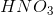and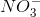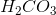and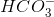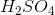and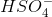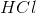and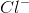andExplanation:

In this question, we're presented with a variety of acid/base pairs and we're asked to identify which one could act as a buffer.

Remember that a buffer is a pair of acid and its conjugate base that acts to resist substantial changes in pH. In order for a buffer to work, the acid base pair needs to exist in equilibrium. This way, when the pH of the solution changes, the equilibrium of the acid/base reaction will shift, such that the pH will not change drastically.

To have an acid/base pair in equilibrium, we'll need to look for a pair that contains a weak acid. Acids likeandare so strong that they will dissociate completely. Of the answer choices shown, only the carbonic acid/bicarbonate system (and) exists in equilibrium. Thus, this is the correct answer.

### Example Question #2 : Buffers

You are in chemistry lab performing a titration. You were given 15 mL of an aqueous solution with an unknown concentration of acetic acid,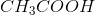to solve through titration with concentrated sodium hydroxide,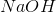. You know that the pKa of acetic acid is 4.75 and that your titrant is 0.1 M sodium hydroxide,The endpoint was determined at 10 mL of sodium hydroxide,. What is the pH after 5 mL ofwas added?

8.45

9.25

4.75

7

4.75

Explanation:

At the half end point, the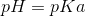. This can be determined by the Henderson-Hasselbalch equation if it is not clear.

Since the endpoint of the titration is that there are 10 mL of 0.1 M NaOH added, that means that there are 0.001 moles of acetic acid.

When 5 mL of NaOH is added, there are 0.0005 moles of acetic acid and 0.0005 moles of acetate formed.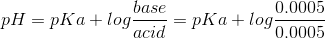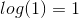Therefore pH= pKa

### Example Question #3 : Buffers

Determine which combination of solutions would create a buffer solution.

10 mL of 0.4 M NaOH + 10 mL of 0.2 M ammonium

50 mL of 0.4 M NaOH + 50 mL of 0.8 M ammonium

5 mL of 0.4 M NaOH + 10 mL of 0.2 M ammonium

25 mL of 0.4 M NaOH + 50 mL of 0.2 M ammonium

50 mL of 0.4 M NaOH + 50 mL of 0.8 M ammonium

Explanation:

For all the other options there is no ammonium leftover with which to serve as the weak acid in the buffer system, the ammonium is all used up and converted to ammonia. However in the correct answer choice, there is enough ammonium leftover after the reaction with the sodium hydroxide.

### Example Question #4 : Buffers

Determine the pH of an aqueous solution of 0.01 M acetic acid,. The pKa of acetic acid is 4.75.

0.161

7

2.87

3.45

2.87

Explanation:

Since acetic acid is a weak acid, it has a Ka that is rather small, we have to do a RICE table to determine the equilibrium amount of hydronium, H3O+ to then determine the pH.

R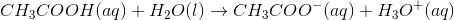I           0.1 M              -                 0                      0

C           -x                                    +x                   +x

E          0.1 -x                                 x                      x

So first we need to change our pKa to a Ka

where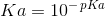therefore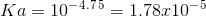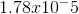=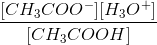=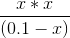If we assume that x is very small compared to 0.1...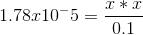Where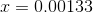(note: when solving using the quadratic we come up with the same answer)

So if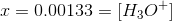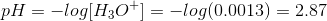### Example Question #5 : Buffers

Determine which solution(s) will yield a buffer solution.

I. 10 mL of 0.5 M HCl + 20 mL of 0.5 M acetate

II. 10 mL of 0.5 M HCl + 10 mL of 0.5 M acetate

III. 10 mL of 0.5 M HCl + 10 mL of 1.0 M acetate

IV. 10 mL of 0.5 M HCl + 10 mL of 1.5 M acetate

I, III, and IV

I, II, III, and IV

I only

III and IV

I and III

I, III, and IV

Explanation:

These answers are correct because the two components needed to create a buffer solution are a weak acid and its conjugate base, or a weak base and its conjugate acid. In these cases, the first reaction to occur upon addition of the strong acid is the formation of the conjugate acid, acetic acid.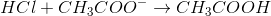If the amount of initial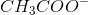is greater than HCl, then we will have someleft over to act as a buffer with the created conjugate acid. This can be through a greater volume, or through a higher concentration as shown in the correct answers.

### Example Question #6 : Buffers

Which combination(s) would create a buffer solution?

I. Weak acid

II. Weak acid's conjugate base

III. Strong acid

IV. Strong base

V. Weak base

VI. Weak base's conjugate acid

I and II; V and VI

None of these will combine to form a buffer solution

I and II; III and IV; V and VI

III and IV only

I and II; V and VI

Explanation:

A buffer solution is formed from the equilibrium of a weak acid and its conjugate base, or from a weak base and its conjugate acid. It's ability to "buffer" the pH or keep it from changing in large amounts in from the switching between these two forms weak and its conjugate.

### Example Question #7 : Buffers

Determine which of these solution combinations form a buffer.

10 mL of 0.5 M NaOH + 10 mL of 0.5 M HCl

10 mL of 0.5 M NaOH + 20 mL of 0.5 M ammonium

10 mL of 0.5 M NaOH + 10 mL of 0.5 M ammonia

10 mL of 0.5 M NaOH + 10 mL of 0.5 M ammonium

10 mL of 0.5 M NaOH + 20 mL of 0.5 M ammonium

Explanation:

First to go through why the other ones are wrong:

Strong base + strong acid neutralizes and does not form a buffer solution

Strong base + weak base does not form a buffer - would need an acid

Strong base + weak acid  = all weak acid converted to conjugate base